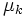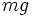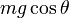# Friction

View a complete list of force types

## Definition

### Intensional definition

Friction (sometimes called sliding friction to distinguish from rolling friction) is the component of contact force between two bodies with a surface of contact acting in a direction along (or parallel to) the surface of contact, or the tangent plane through the point of contact.

The term friction refers by default to dry friction: friction where the two surfaces of contact are dry and solid. The typical model used for such friction in classical mechanics is the Coulomb model of friction. More complicated models that take into account the many causal mechanisms of friction are studied in tribology.

### Tendency-based definition

Friction refers to a force exerted by two bodies on each other, through a surface of contact, that tends to oppose slipping of the two surfaces of contact against each other. Note that static friction opposes the tendency indicated by the direction of net external force along the plane of contact, while kinetic friction opposes the direction of actual motion.

## Situational examples

Here, where there are only two surfaces in contact, we denote by$\mu_s$ the limiting coefficient of static friction and by$\mu_k$ the coefficient of kinetic friction.$g$ is the acceleration due to gravity.

Example Kind of friction (static versus kinetic) Direction of friction force on each body Magnitude of friction force Reason
Block placed on a fixed horizontal plane, no external applied forces No friction No direction since no friction 0 Friction arises only to oppose a slipping tendency. When there is no such tendency, no friction arises.
Block placed on a fixed horizontal plane. A small horizontal push is applied to the block but fails to move the block. Static friction Opposite to the horizontal direction of applied force on the block; along the direction of the applied force on the fixed plane. Equal to the magnitude of the horizontal applied force Since the body does not move, the friction force must exactly counterbalance the applied force.
Block sliding along a fixed horizontal plane, no external applied forces Kinetic friction Opposite direction of motion of block on block, along direction of motion of block on the plane$\mu_kmg$ where$m$ is the mass of the block The normal force equals$mg$
Block sliding along a fixed horizontal plane, external applied force in a horizontal direction opposite to the direction of sliding. Kinetic friction Opposite direction of motion of block on block, along direction of motion of block on plane.$\mu_kmg$ where$m$ is the mass of the block The normal force equals$mg$. Note that the other external applied force plays no role in determining the magnitude of the friction force because when a body is moving, friction opposes the direction of motion and not the direction of external applied force.
Block placed on another block which is on a fixed horizontal surface. The lower block is being accelerated horizontally and the upper block moves along with it without slipping. No other applied force on the block on top. Static friction Along the direction of acceleration for the block on top. Opposite the direction of acceleration for the block at the bottom. The mass of the block on top times the achieved acceleration. The friction force is the force solely responsible for keeping the block on top accelerating along with the block on the bottom.
Block sliding along an inclined plane, angle$\theta$ with horizontal, no external applied forces Kinetic friction Opposite direction of motion of block on block, along direction of motion of block on surface$\mu_kmg \cos \theta$ where$m$ is the mass of the block The normal force equals$mg \cos \theta$

## Related forces

### Types of friction

• Static friction: This is friction exerted when the surfaces of contact are not slipping relative to each other. This occurs, for instance, both when the surfaces are stationary, and in the case of rolling. Static friction acts to precisely cancel the net external force along the plane of contact. According to the Coulomb model of friction, the maximum possible value of static friction is$\mu_sN$ where$N$ is the normal force and$\mu_s$ is the limiting coefficient of static friction for the two surfaces.
• Kinetic friction or dynamic friction: This is friction exerted when the surfaces are slipping relative to each other. Kinetic friction acts in the direction opposite to the direction of slipping. According to the Coulomb model of friction, for small relative velocities, its magnitude is$\mu_kN$, where$N$ is the normal force and$\mu_k$ is the coefficient of kinetic friction.

### Forces between rigid bodies

• Rolling friction: This is not really friction, but rather, a normal force that effectively plays the role of friction due to local deformations of the surface.
• Normal force: The component of contact force in the direction perpendicular to the plane of contact.